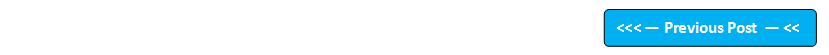Google+

## Blog Pages

### 240. Using Programmatic Method instead of Exception HandlingWe have to programmatically check for expected errors before going for any exception handling technique.

For example -

int a=9,b=0,x;
int x = a/b;  //Gives arithmetic exception

We can handle the above exception using the try catch block as shown below -

try {
int a=9,b=0,x;
int x = a/b;  //Gives arithmetic exception
}
catch(ArithemeticException e) {
e.printStackTrace( ); //Handles the exception and prints details
}

We can also handle the divide by zero error programmatically  by using the if condition in our program instead of handling the exception using try catch blocks as shown below -

if(b==0)
System.out.println("The value of b cannot be zero");
else
int x = a/b;

Hence if we know that an exception is going to occur in our code, we have to think of programmatically resolving the error. If we are not able to programmatically resolve the error say 'Find Not Found' etc., then only we have to go for try catch blocks.

Lets implement this concept on Eclipse IDE -

1. Launch Eclipse IDE, create a Java Class 'ProgramHandling.java' with main( ) method in the existing Java Project 'Project 47' as shown below -

2. Create a try catch block to handle any arithmetic exception which can be programmatically handled as shown below -

3. Save & Run the Java Class 'ProgramHandling.java' and observe that the exception is handled and the statement after the catch block is executed as shown in the below output -

4. Now lets programmatically check for the expected arithmetic exception by using if else to resolve the program without actually using the exception handling method  -

5. Save & Run the Java Class 'ProgramHandling.java' and observe that the error is handled by the if else blocks in the program code as shown in the below output -

Hence whenever we get a change to resolve the error programmatically, use programmatic method instead of exception handling techniques like try catch block. Use exception handling techniques when you are not able to handle the errors in the code using programmatic method.

Please comment below to feedback or ask questions.